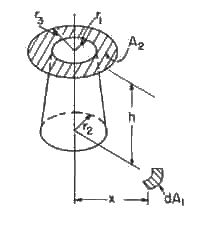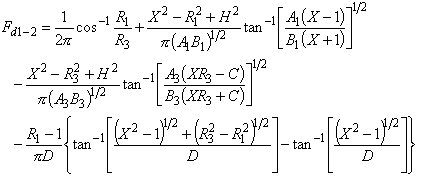>

# SECTION B Factors From Differential Elements to Finite Areas

## B-27: Plane element to annular disk with conical blockage of view.Definitions:

 X=x/r2 A=(X+R)2+H2 R1=r1/r2 B=(X-R)2+H2 H=h/r2 C=R1-[(X2-1)(R32- R12)]1/2 R3=r3/r2 D=[(R1-1)2+H2]1/2

Governing equation:To download a copy of this equation in MS Word 97 Format, click HERE.